Close

# Defining prior distributions for sensitivity/specificity in the latent class model with random effects

This blog post describes how to set informative prior distributions for the sensitivity or specificity parameters in the latent class model with random effects described in the article by Dendukuri and Joseph(2001). Though the text below refers to the sensitivity, it applies equally to the specificity.

### Visualizing the distribution of sensitivity

Under the random effects model each subject is assumed to have a different sensitivity. The sensitivity of the ith individual is expressed as $$\Phi(a+br_i)$$ where $$a$$ and $$b$$ are unknown parameters for which a prior distribution needs to be provided, and where $$r_i$$ is a random effect following a standard normal distribution $$r_i \sim N(0,1)$$. $$\Phi$$ denotes the cumulative probability of the standard normal distribution. For simplicity the subscript $$i$$ of the random effect is suppressed and it is written as $$r$$ in the rest of this post. It can be shown that the marginal (or average) sensitivity, across all values of $$r$$, is $$\Phi(\frac{a}{\sqrt{1+b^2}})$$.

Consider the case when $$a=1$$ and $$b=0.5$$. Then the relation between the sensitivity of the ith individual and $$r$$ would look like this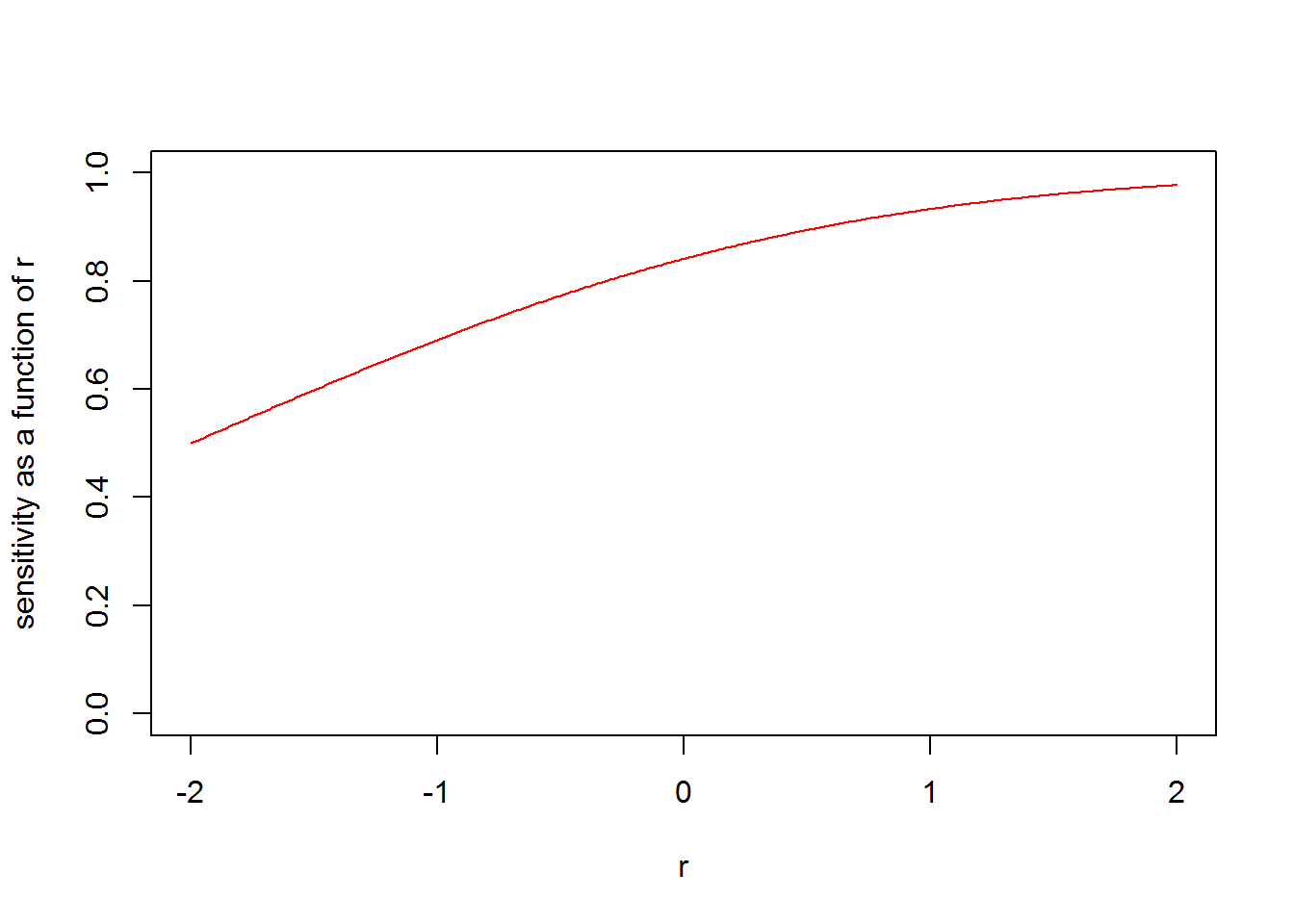In this case the marginal sensitivity $$\Phi(\frac{a}{\sqrt{1+b^2}})$$ is 0.81. We can see from the plot above that individual sensitivities range from about 0.5 to 0.95. The sensitivity for an individual with $$r$$=0 is $$\Phi(1)$$=0.84 which is close to the marginal sensitivity.

From the plot below we can see that if $$b$$ were 0 then the sensitivity would be the same for all subjects. As $$b$$ increases the the variation in sensitivity increases.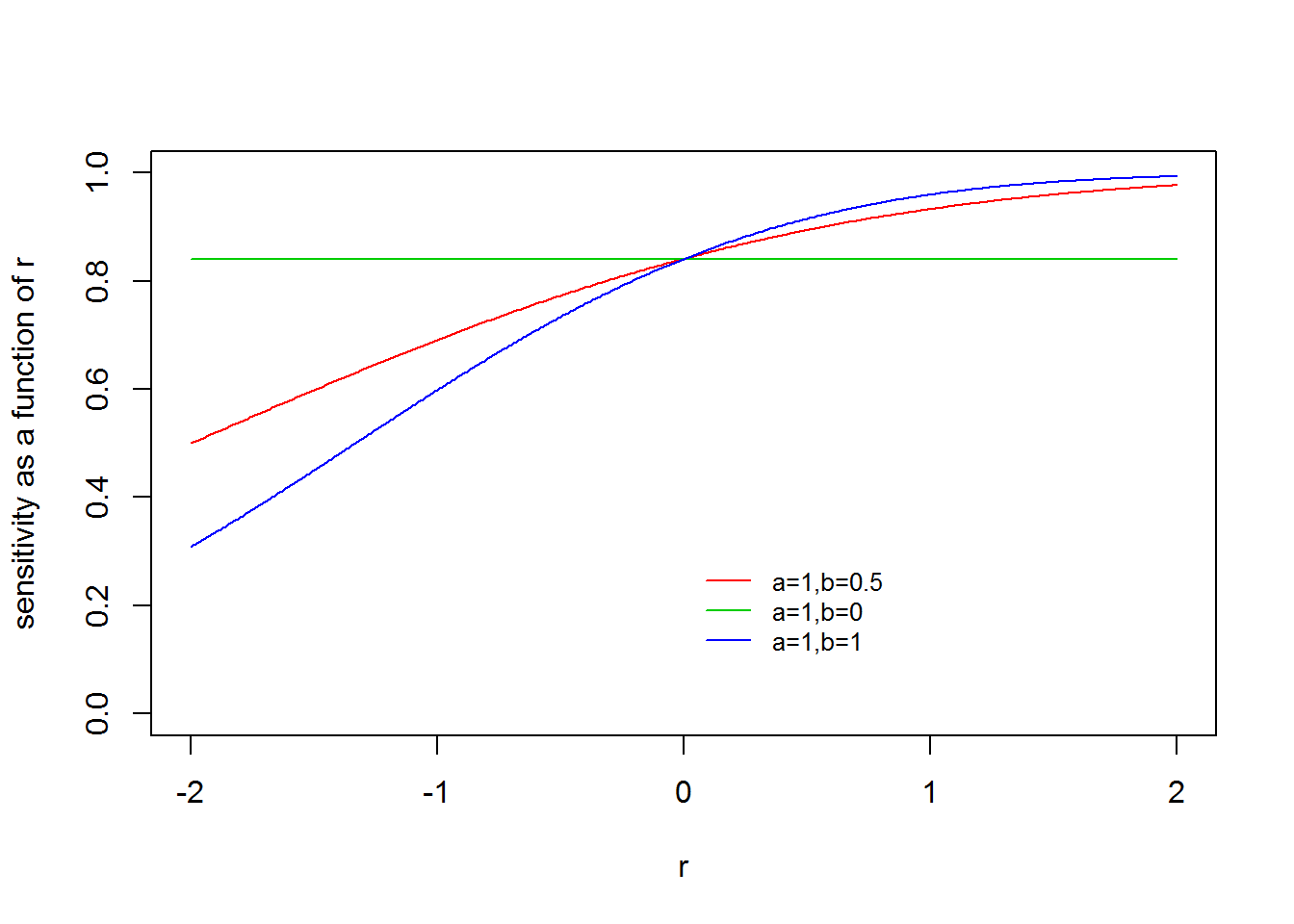Thus $$a$$ has greater influence on the marginal sensitivity while $$b$$ exerts influence on the range of sensitivity across individuals.

## Prior information

We need to specify a prior distribution for $$a$$ and one for $$b$$. Prior information is typically available on the marginal sensitivity, which should be enough to determine the prior distribution on $$a$$. But we would need an additional source of prior information to determine the prior information for $$b$$. In the original article, we had used subjective information on the covariance between two tests. Another possibility is the range of sensitivity across individuals in the population. In this note we focus on the latter type of prior information as it is computationally easier to determine the prior distribution of $$b$$.

In practice it is not always possible to observe the range of sensitivity of a test across a population because we do not always know of the underlying variable(s) that affect the sensitivity of a test. Indeed, this is why we are using a random effect to represent the impact of these unmeasured underlying variables. In some cases, it may be possible to elicit this information from a subject expert. In other cases we may have knowledge of the key variable(s) that are responsible for causing a variation in the sensitivity.

For example, it is known that smear microscopy status is an important determinant of the sensitivity of the Xpert test for pulmonary tuberculosis in adults. A recent meta-analysis by Steingart et al(2014) of the accuracy of the Xpert MTB/RIF assay for pulmonary TB in adults reported that among the sub-group of smear negative individuals the 95% credible interval for the pooled sensitivity was (60%, 74%). On the other hand, among smear positive individuals it was (97%, 99%). Using the end-points of these two credible intervals, we may make the subjective assumption that for 95% of individuals sensitivity of Xpert ranges between 60% and 99%. The same meta-analysis reported that a 95% credible interval for the pooled sensitivity overall (i.e. including smear positive and smear negative patients) was (85%, 92%).

## Translating prior information into prior distributions

We assume that the parameter $$a$$ follows a normal prior distribution with mean $$\mu_{a}$$ and standard deviation $$\sigma_{a}$$. The parameter $$b$$ is assumed to be independent of $$a$$, and is assumed to follow a normal prior distribution with mean $$\mu_b$$=0 and standard deviation $$\sigma_{b}$$. We use a symmetric distribution for $$b$$ about 0 to allow the sensitivity to be either positively or negatively correlated with the random effect. Let (LC, UC) denote the 95% credible interval and (LP, UP) denote the 95% prediction interval.

To determine the prior distribution on $$a$$ we match the ends of the 95% credible interval from the meta-analysis to the expressions $$\Phi(\mu_{a} \pm 1.96\sigma_{a})$$. This yields $$\mu_{a}=(\Phi$$-1(UC)+$$\Phi$$-1(LC))/2 and $$\sigma_{a}=(\Phi$$-1(UC)-$$\Phi$$-1(LC))/4.

In order to determine $$\sigma_b$$ Note that Variance($$a+br$$) = Variance($$a$$)+Variance($$b$$)$$r^2=\sigma_a^2+r^2\sigma_b^2$$, as $$r$$ is treated as a constant. To determine the value of $$\sigma_{b}$$ we match the upper 95% limit of the individual sensitivity to $$\Phi(\mu_{a}+1.96 \sqrt{\sigma_a^2+r^2\sigma_b^2)}$$. Alternatively, we could match the lower 95% limit of the individual sensitivity to $$\Phi(\mu_{a}-1.96 \sqrt{\sigma_a^2+r^2\sigma_b^2)}$$. This implies

$\sigma_{b}=max(\frac{\sqrt{((\Phi^{-1}(UP)-\mu_{a} )/1.96)^2 – \sigma_{a}^2}}{1.96},\frac{\sqrt{((\mu_{a}-\Phi^{-1}(LP))/1.96)^2 – \sigma_{a}^2}}{1.96})$.

### Example

We now apply the expressions above to determine the prior distributions for $$a$$ and $$b$$ in the context of the Xpert test. The parameters for the prior distribution of $$a$$ are determined using the following R code

LC=0.85
UC=0.92
mu_a=(qnorm(UC)+qnorm(LC))/2
sigma_a=(qnorm(UC)-qnorm(LC))/4

The parameters for the prior distribution of $$b$$ are determined using the following R code

LP=0.60
UP=0.99
sigma_b= max(sqrt(((qnorm(UP)-mu_a)/1.96)^2 - sigma_a^2)/1.96,sqrt(((mu_a-qnorm(LP))/1.96)^2 - sigma_a^2)/1.96)

The figure below illustrates the histograms of $$a$$ and $$b$$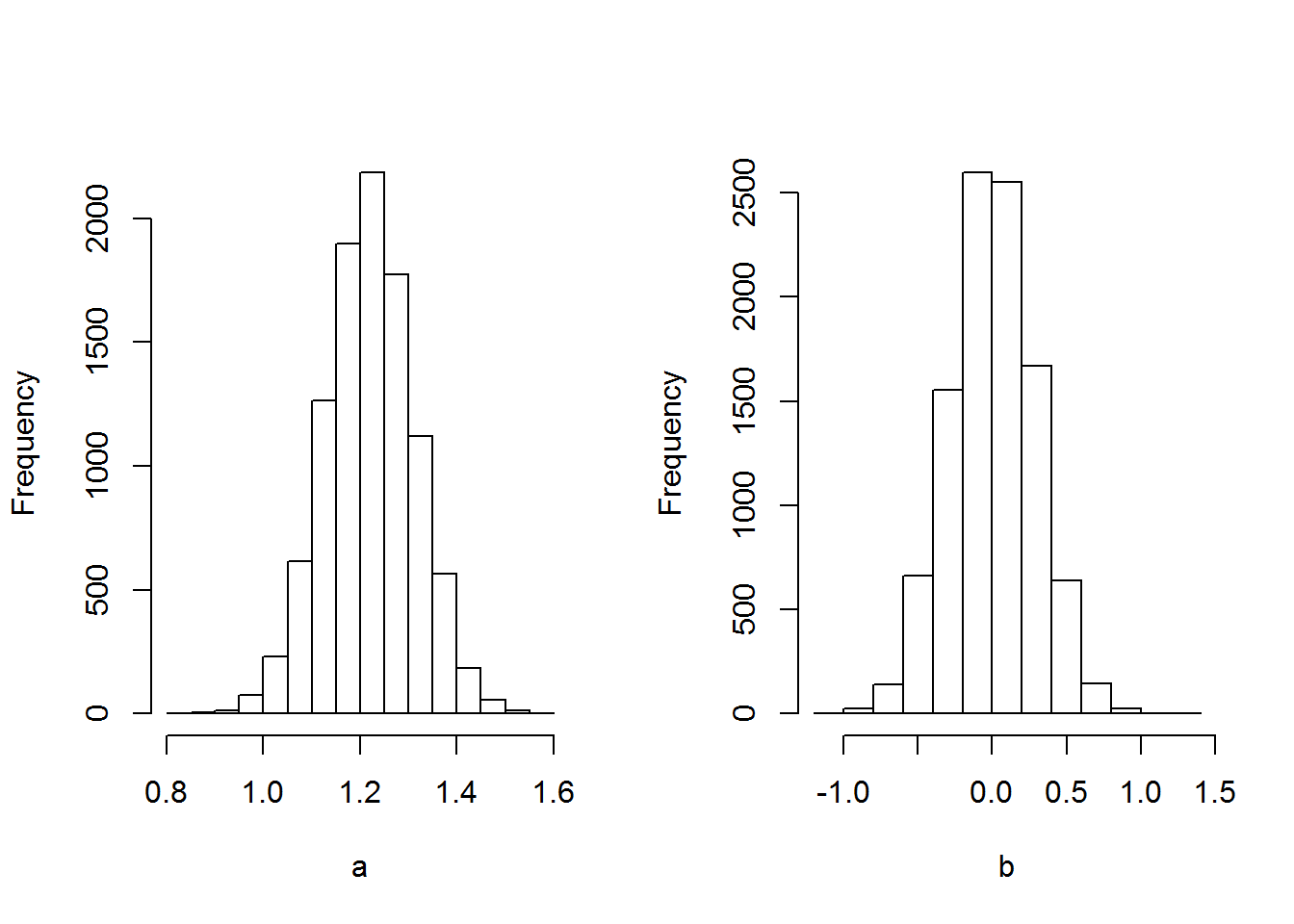The figure below illustrates the histograms of: i) the median sensitivity (when $$r$$=0), ii) the marginal sensitivity, iii) the individual sensitivity when $$r$$=1.96 and iv) the individual sensitivity when $$r$$=0.67.

We can see that the 2.5%ile and 97.5%iles (red lines) of the distributions of the mean and median sensitivity roughly match the limits of the 95% credible interval. It should noted that the histogram for the case when $$r$$=1.96 is also the histogram for the case $$r$$=-1.96 due to the symmetric distribution of $$b$$. The 2.5%ile and 97.5%ile of the distribution of the individual sensitivity when $$r$$=1.96 roughly match the limits of the subjective 95% interval we had provided for the individual sensitivities.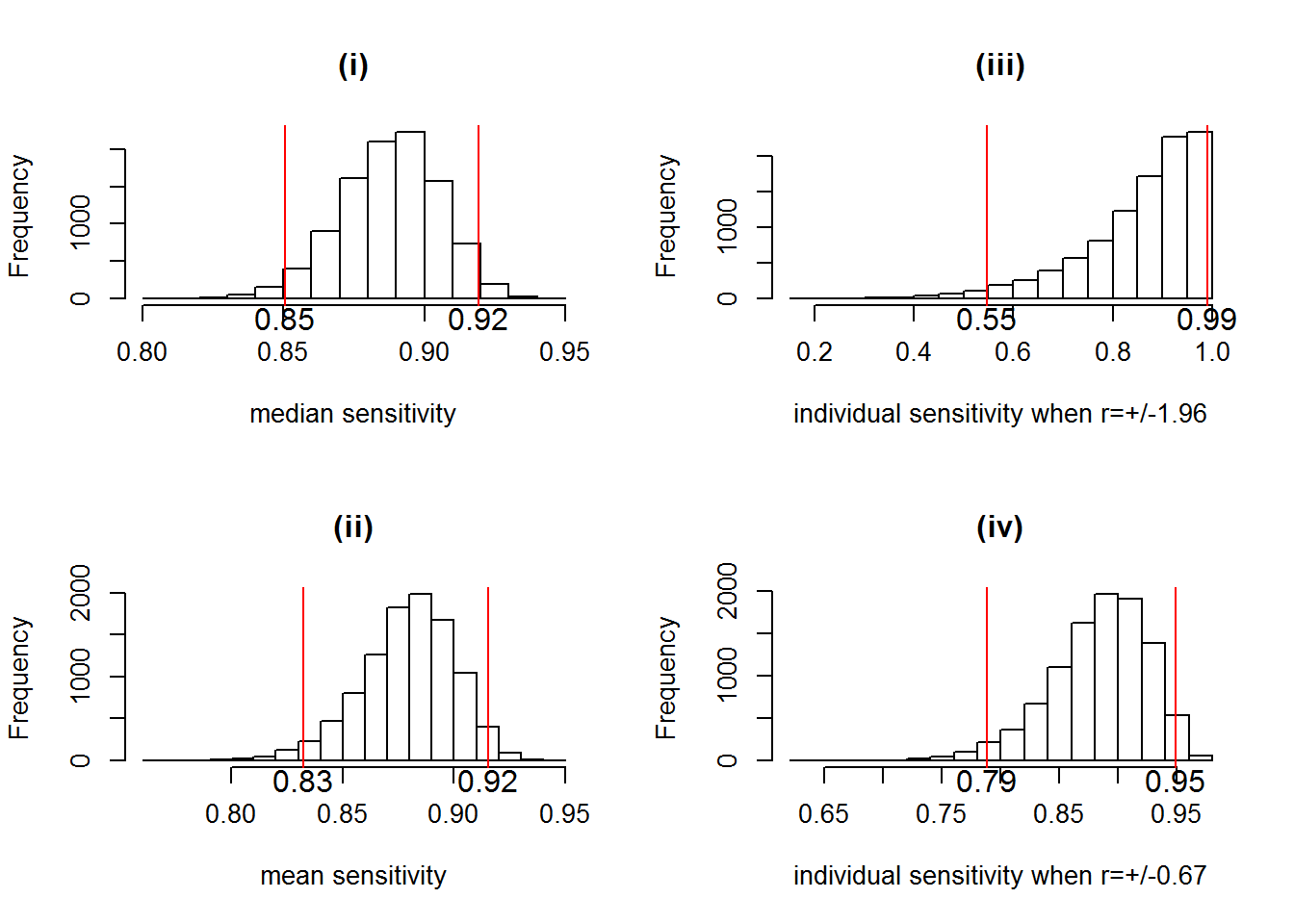## Comparison to our previous approach

In the original paper by Dendukuri and Joseph(2001) we had used a different approach based on a bisectional search for the prior distribution parameters of two tests and the covariance between them. Therefore the results obtained with the method above will not exactly match what was reported in that paper, particularly for the $$b$$ parameter.

There as well we had remarked that the prior information available on the marginal sensitivity primarily served to determine the prior distribution for $$a$$. The prior standard deviation of $$b$$ was selected somewhat arbitrarily as no prior additional information was available. Here we have shown that if additional prior information is available in terms of a 95% interval covering the sensitivities of individual subjects, that could be used to determine $$\sigma_b$$.

For the Strongyloides example reported on in that paper, the range of prior information over the marginal sensitivity of microscopy was (7%, 47%). In the absence of any prior information on the individual sensitivities we could use a very wide range from (0.1%, 99.9%). This is equivalent to saying that there are some individuals in whom the test has nearly 0% sensitivity and at the other extreme there are patients in whom it has a 100% sensitivity. It is possible that such a gradation is created by the severity of infection. Patients with a mild infection may have a very low bacterial load that is not detectable by microscopy and those with a severe infection and correspondingly high bacterial load are easily detected. Using the method above, this would correspond to

LC=0.07
UC=0.47
mu_a=(qnorm(UC)+qnorm(LC))/2
sigma_a=(qnorm(UC)-qnorm(LC))/4
print(mu_a)
##  -0.7755
print(sigma_a)
##  0.3501

The parameters for the prior distribution of $$b$$ are determined using the following R code

LP=0.001
UP=0.999
sigma_b= max(sqrt(((qnorm(UP)-mu_a)/1.96)^2 - sigma_a^2)/1.96,sqrt(((mu_a-qnorm(LP))/1.96)^2 - sigma_a^2)/1.96)
print(sigma_b)
##  0.9903

The prior distribution over $$a$$ remains roughly the same as in the original paper where $$\mu_a=-0.811$$ and $$\sigma_a=0.380$$, but the prior distribution over $$b$$ is now less informative compared to the original paper where $$\mu_b=0.668$$ and $$\sigma_b=0.5$$. The resulting histograms over the marginal and individual sensitivities are given below. Notice how the very wide prior on $$b$$ results in placing more weight on values of the individual sensitivity close to 0 or 1.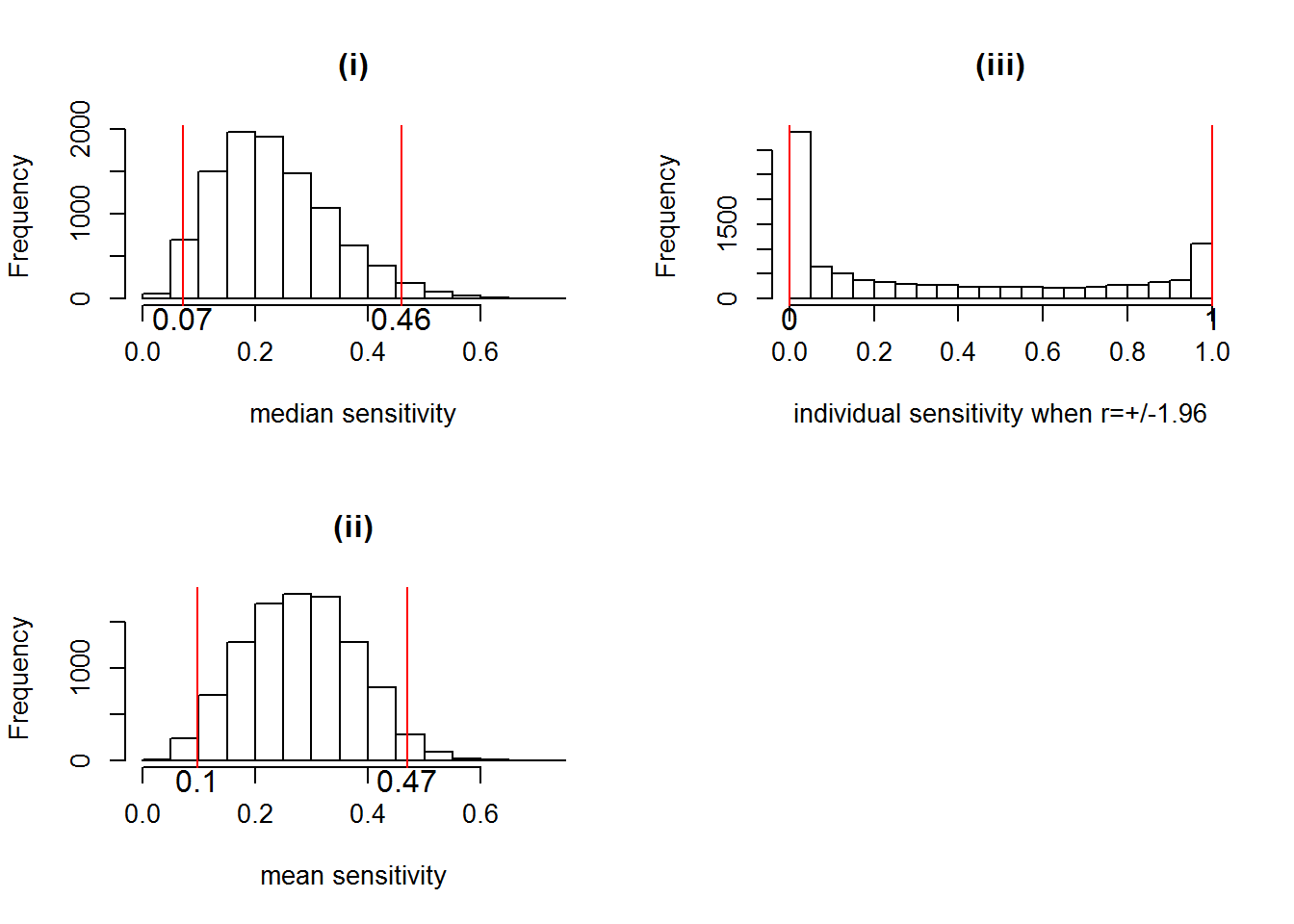## References

Dendukuri, Nandini, and Lawrence Joseph. 2001. “Bayesian Approaches to Modeling the Conditional Dependence Between Multiple Diagnostic Tests.” Biometrics, no. March: 158–67. http://www.jstor.org/stable/2676854.

Steingart, KR, I Schiller, DJ Horne, M Pai, Catharina C. Boehme, and N Dendukuri. 2014. “Xpert® MTB/RIF Assay for Pulmonary Tuberculosis and Rifampicin Resistance in Adults.” Cochrane Database Syst Rev, no. 1,CD009593. http://onlinelibrary.wiley.com/doi/10.1002/14651858.CD009593.pub3/epdf/standard.